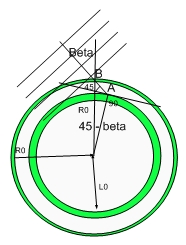# Hypergeometrical Universe

## The Beginning of Times

From time immemorial we have been asking Why?

Why might be a tall order to explain.  I might be able to tell you how?...:)

The main characteristic of our lives is causality.  You write a blog in the morning, and you might be late to work..:) (so I will be fast..)

Causality means that current events are connected to past events through some action. In physics, that action is actual action-at-distance....Gravitation, Electromagnetism are forces which act at distance. Notice that I didn't mention the others (Electro-weak or Strong).  They play no role in my theory. Anything that acts at distance implies that you are interacting with the past.  It takes time for the field (dilaton field) to reach you.

There is an Anthropic Principle (which vaguely states as an answer for the why the Universe is like this - It is like this otherwise we couldn't ask this question right now and here..:) - a circular reasoning not unlike my hypergeometrical universe cross-sections...:)

The question I will answer is if there is a deeper understanding we can derive from the Hypergeometrical Universe theory that would provide an answer to that question with a little more details.

This picture has been used to explain how we peer into the past with our telescopes.  It also can be used to explain the Universe is causal and why we are the way we are...:)

Ooops, I guess I will explain the WHY after all..:)

Consider a point in a four dimensional spatial manifold.  Locally deform the metric such that the total 4D volume surrounding the deformation is preserved. The initial deformational mode is debatable (Y00, Y01, TEM00..::)  Now, allow for that deformation to decay into a gazillion spinning smaller deformations.

I used spinning instead of rotating to emphasize the fact that the smaller metric deformation (spinning deformation coherences) are rotating within a 4D spatial manifold as opposed to our 3D rotating objects.

Some of them will annihilate each other resulting in a locally flat metric. The annihilation  (not unlike a eletron-positron annihilation) will generate dilatons (Gamma Radiation) which carry momentum.

Now let's consider that the dilaton-dilator interaction depends upon cross-sections, that is, how are the dilators aligned at time zero.  Only the dilators which are perfectly aligned with their largest cross-sections perpendicular to R (the 4D radial direction) will be pushed away by the extremely intense dilaton field. This is the synchronization or selection event.  Further interactions might contain a mechanism to resynchronize  (select) dilators as the Universe travels.

I mentioned R, but at time zero, R,Z,Y,Z are indistinguishable, thus one would expect that dilators would be flying at all directions with identical speed( the maximum possible while driven by an extremely intense dilaton field).  I will postulate that the whole Universe contained in a lightspeed expanding hyperspherical shockwave shell reached the speed of light.  This postulate will be immediately tested later in this blog when we consider the nonexistence of interaction or visibility cut-offs in this Universe.

Add to that picture the Quantum Lagrangian Principle and you will have an event that accelerated equally (to our observed c) all dilators in all directions (XYZR).

That scenario would correspond to all matter in the Universe being spin synchronized and accelerated to c at time zero.Notice that at time zero, the very small hypersphere has interaction with a very close past and the dilaton field is hyper intense.  At that level, dilators are capable of reaching the speed of light in a single or couple of de Broglie steps.

The interaction with the past should deliver the maximum kinetic energy while allowing for the dilators to surf the dilaton field.  The condition where this happens is when the average dilator interaction happens at 45 degrees, that is the shockwave hypersphere has been accelerated to the observed speed of light, which is the same as saying that the tangential speed (along X or Y or Z) is equal to the speed of light. Both are true since there isn't a difference between tangential speed and radial speed for a point.

Being spin synchronized means that we are all here interacting intermittently (as the dilators spin), living in a thin hypersphere, always interacting with our past at 45 degrees (thus preserving the constancy of the speed of light.  This angle corresponds to the maximum interaction and the equipartition of energy along all four degrees of freedom (spatial coordinates) at time zero..

Imagine otherwise that we were accelerated radially at higher speed that laterally.  This would mean that all forces would not vanish with the inverse of the square distance but just have a cut-off...  Causality would be space dependent.  We would be blind to anything further than that cut-off distance.

Thus the WHY is explained with simple geometrical reasoning based on cross-sections of dilators to dilaton interaction at the Beginning of Times. That yielded the dilator spin synchronization and the intermittently interacting Universe (Quantum Mechanics).  Of course, QM also depends upon the QLP (Quantum Lagrangian Principle) and the inherent delocalization of dilators traveling in an out of a 3D hypersurface guided by dilaton interference patterns (three dimensional Lissajous pattern).

As you can see, if the Big Bang wasn't really Big (accerating everything to the maximum velocity) and if the Bang wasn't really isotropic (along all four spatial dimensions) we wouldn't have Cosmological Causal Relationships today.  The night sky would be darker and we wouldn't be allowed to see beyond a point in the Cosmos - Cosmic Censorship...:) of the worse kind.

The isotropy (Michelson Morley) of the speed of light is the direct result of the initial isotropic acceleration.
The causality is because we interact perfectly at 45 degrees with the past hypersphere, thus creating a no cut-off interaction....:)

Thus permitting us to stand in a cloudless night and watch the whole Universe ...

Cheers,

MP
Currently unrated

or